# Beta decay

Nuclear physics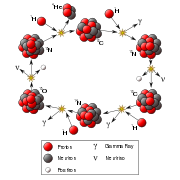Nuclear fission
Nuclear fusion

In nuclear physics, beta decay is a type of radioactive decay involving the emission of beta particles. Beta particles are high-energy, high-speed electrons or positrons emitted by certain types of radioactive atomic nuclei such as potassium-40. These particles, designated by the Greek letter beta (β), are a form of ionizing radiation and are also known as beta rays.

There are two forms of beta decay: "beta minus" (β), involving the release of electrons; and "beta plus" (β+), involving the emission of positrons (which are antiparticles of electrons). In beta minus decay, a neutron is converted into a proton, an electron, and an electron antineutrino. In beta plus decay, a proton is converted into a neutron, a positron, and an electron neutrino (a type of neutrino associated with the electron). In either case, the number of nucleons (neutrons plus protons) in the nucleus remains the same, while the number of protons in the nucleus changes.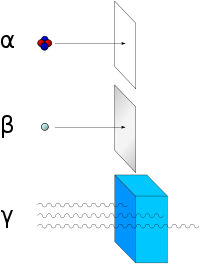Alpha radiation consists of helium nuclei and is readily stopped by a sheet of paper. Beta radiation, consisting of electrons, is halted by an aluminum plate. Gamma radiation is eventually absorbed as it penetrates a dense material.

If the atomic nuclei of a chemical element undergo beta decay, this process leads to the transmutation of that element into another. It is one way by which unstable atomic nuclei acquire greater stability. Beta minus decay is a common process in the neutron-rich fission by-products produced in nuclear reactors, accounting for the large numbers of electron antineutrinos produced by these reactors. Free neutrons also decay by this process.

## History

Historically, the study of beta decay provided the first physical evidence of the neutrino. In 1911, Lise Meitner and Otto Hahn performed an experiment that showed that the energies of electrons emitted by beta decay had a continuous rather than discrete spectrum. This was in apparent contradiction to the law of conservation of energy, as it appeared that energy was lost in the beta decay process. A second problem was that the spin of the Nitrogen-14 atom was 1, in contradiction to the Rutherford prediction of ½.

In 1920-1927, Charles Drummond Ellis (along with James Chadwick and colleagues) established clearly that the beta decay spectrum really is continuous, ending all controversies.

In a famous letter written in 1930, Wolfgang Pauli suggested that in addition to electrons and protons atoms also contained an extremely light neutral particle which he called the neutron. He suggested that this "neutron" was also emitted during beta decay and had simply not yet been observed. In 1931, Enrico Fermi renamed Pauli's "neutron" to neutrino, and in 1934 Fermi published a very successful model of beta decay in which neutrinos were produced.

## β− decay (electron emission)

An unstable atomic nucleus with an excess of neutrons may undergo β decay. In this process, a neutron is converted into a proton, an electron, and an electron-type antineutrino (the antiparticle of the neutrino):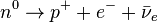$n^0 \rightarrow p^+ + e^- + \bar{\nu}_e$.

At the fundamental level (depicted in the Feynman diagram below), this process is mediated by the weak interaction. A neutron (one up quark and two down quarks) turns into a proton (two up quarks and one down quark) by the conversion of a down quark to an up quark, with the emission of a W- boson. The W- boson subsequently decays into an electron and an antineutrino.

Beta decay commonly occurs among the neutron-rich fission byproducts produced in nuclear reactors. This process is the source of the large numbers of electron antineutrinos produced by fission reactors. Free neutrons also decay via this process.

## β+ decay (positron emission)

Unstable atomic nuclei with an excess of protons may undergo β+ decay, or inverse beta decay. In this case, energy is used to convert a proton into a neutron, a positron (e+), and an electron-type neutrino ($\nu_e$):$\mathrm{energy} + p^+ \rightarrow n^0 + e^+ + {\nu}_e$.

On a fundamental level, an up quark is converted into a down quark, emitting a W+ boson that then decays into a positron and a neutrino.

Unlike beta minus decay, beta plus decay cannot occur in isolation, because it requires energy — the mass of the neutron being greater than the mass of the proton. Beta plus decay can only happen inside nuclei when the absolute value of the binding energy of the daughter nucleus is higher than that of the mother nucleus. The difference between these energies goes into the reaction of converting a proton into a neutron, a positron and, a neutrino and into the kinetic energy of these particles.

## Electron capture

(See main article on Electron capture.)

In all cases where β+ decay is allowed energetically (and the proton is part of an atomic nucleus surrounded by electron shells), it is accompanied by the "electron capture" process, also known as inverse beta decay. In this process, a proton in the atomic nucleus captures an atomic electron (from an inner orbital), with the emission of a neutrino. The proton is converted into a neutron. The process may be written as follows:$\mathrm{energy} + p^+ + e^- \rightarrow n^0 + {\nu}_e$.

If, however, the energy difference between initial and final states is low (less than 2mec2), then β+ decay is not energetically possible, and electron capture is the sole decay mode.

## Effects of beta decay

Beta decay does not change the number of nucleons A in the nucleus, but changes only its charge Z. Thus, during beta decay, the parent nuclide and daughter nuclide share the same A value.

The beta decay of atomic nuclei results in the transmutation of one chemical element into another. For example:

• Beta minus: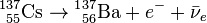$\mathrm{{}^1{}^{37}_{55}Cs}\rightarrow\mathrm{{}^1{}^{37}_{56}Ba}+ e^- + \bar{\nu}_e$
• Beta plus: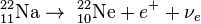$\mathrm{~^{22}_{11}Na}\rightarrow\mathrm{~^{22}_{10}Ne} + e^+ + {\nu}_e$

For comparison, the electron capture process may be written as follows:

• Electron capture: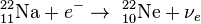$\mathrm{~^{22}_{11}Na} + e^- \rightarrow\mathrm{~^{22}_{10}Ne} + {\nu}_e$

In nature, most isotopes are beta stable, but a few exceptions exist with half-lives so long that they have not had enough time to decay since the moment of their nucleosynthesis. One example is 40K, which undergoes beta minus and beta plus decay and electron capture, with a half-life of 1.277×109 years.

It should be noted that a beta-stable nucleus may undergo other kinds of radioactive decay, such as alpha decay.

## Double beta decay

Some nuclei can undergo double beta decay (ββ decay), where the charge of the nucleus changes by two units. In most practically interesting cases, single beta decay is energetically forbidden for such nuclei, because when β and ββ decays are both allowed, the probability of β decay is (usually) much higher, preventing investigations of very rare ββ decays. Thus, ββ decay is usually studied only for beta stable nuclei. Like single beta decay, double beta decay does not change the value of A. Thus, at least one of the nuclides with a given A value has to be stable, for both single and double beta decay.